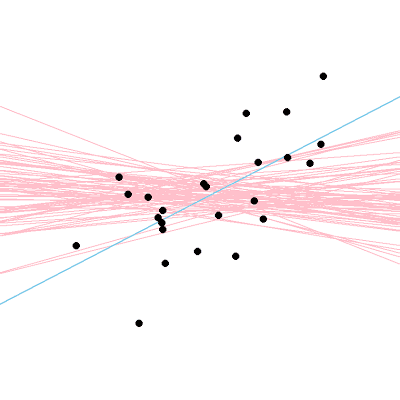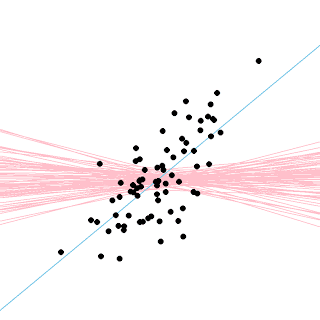## Saturday, December 28, 2013

### Icons for the essence of Bayesian and frequentist data analysis

Goal: Simple graphical icons that capture the essence of Bayesian data analysis and frequentist data analysis. Why? Visual icons serve as mnemonic cognitive packaging that facilitate initial understanding and subsequent remembering. In this post, I propose simple icons that attempt to portray the essence of Bayesian data analysis and frequentist data analysis.

[Feb. 14, 2014: See follow-up post, with new icons, HERE.]

(The prod that got me thinking about this was a light-hearted blog post by Rasmus Baath regarding a "mascot" for Bayesian data analysis. My comment on that post is the beginning of this post. Please note that the icons presented here are not intended as advocacy or cheer leading or as mascots. Instead, I would like the icons to capture succinctly key ideas.)

What is "the essence" of Bayesian data analysis? And what is "the essence" of frequentist data analysis? Any answer will surely provoke disagreement, but that is not my goal. The questions are earnest and often asked by beginners and by experienced practitioners alike. As an educator, I think that the questions deserve earnest answers, with the explicit caveat that they will be incomplete and subject to discussion and improvement. So, here goes.

The essence of Bayesian data analysis is inferring the uncertainty (i.e., relative credibility) of parameters in a model space, given the data. Therefore, an icon should represent the data, the form of the model space, and the credible parameters.

The simplest example I can think of is linear regression: Many people are familiar with x-y scatter plots as diagrams of data, and many people are familiar with lines as a model form. The credible parameters can then be suggested by a smattering of lines sampled from the posterior distribution, like this (Fig. 1):Figure 1. An icon for Bayesian data analysis.
The icon in Figure 1 represents the data as black dots. The icon represents the model form by the obvious linearity of every trend line. The icon represents uncertainty, or relative credibility, by the obvious range of slopes and intercepts, with greater density in the middle of the quiver of lines. I like Figure 1 as a succinct representation of Bayesian analysis because the figure makes it visually obvious that there is a particular model space being considered, and that there is a range of credible possibilities in that space, given the data.

Are there infelicities in Figure 1? Of course. For example, the shape and scale of the noise distribution are not represented. But many ancillary details must be suppressed in any icon.

Perhaps a more important infelicity in Figure 1 is that the prior distribution is not represented, other than the form of the model space. That is, the lines indicate that the prior distribution puts zero probability on quadratic or sinusoidal or other curved trends, but the lines do not indicate the form of the prior distribution on the allowed parameters.

Some people may feel that this lack of representing the prior is a failure to capture an essential part of Bayesian data analysis. Perhaps, therefore, a better icon would include a representation of the prior -- maybe as a smattering of grey lines set behind the data and the blue posterior lines. For the vague prior used in this example, the prior would be a background of randomly criss-crossing grey lines, which might be confusing (and ugly).

Now, an analogous icon for frequentist data analysis.

The essence of frequentist data analysis is inferring the extremity of a data property in the space of possibilities sampled a specified way from a given hypothesis. (That is, inferring a p value.) Note that the data property is often defined with respect to a model family, such as the best fitting slope and intercept in linear regression. Therefore, an icon should represent the data, the data property, and space of possibilities sampled from the hypothesis (with the extremity of the data property revealed by its visual relation to the space of possibilities).

Keeping with the linear regression scenario, an icon for frequentist analysis might look like this (Fig. 2):Figure 2. An icon for frequentist data analysis.
As before, the data are represented by black dots. The data property is represented by the single blue line, which shows the least-squares fit to the data. The space of possibilities is represented by the smattering of red lines, which were created as least-squares fits to randomly resampled x and y values (with replacement, using fixed sample size equal to the data sample size). In other words, the hypothesis here is a "null" hypothesis that there is no systematic covariation between the x and y values. I like Figure 2 as a succinct representation of frequentist data analysis, especially when juxtaposed with Figure 1, because Figure 2 shows that there is a point estimate to describe the data (i.e., the single blue line) and a sample of hypothetical descriptions unrelated to the data.

Are there infelicities in Figure 2? Of course. Perhaps the most obvious is that there is no representation of a confidence interval. In my opinion, to properly represent a confidence interval in the format of Figure 2, there would need to be two additional figures, one for each limit of the confidence interval. One additional figure would show a quiver of red lines generated from the lower limit of the confidence interval, which would show the single blue line among the 2.5% steepest red lines. The second additional figure would show a quiver of red lines generated from the upper limit of confidence interval, which would show the single blue line among the 2.5% shallowest red lines. The key is that there would be a single unchanged blue line in all three figures; what changes across figures is the quiver of red lines sampled from the changing hypothesis.

Well, there you have it. I should have been spending this Saturday morning on a thousand other pressing obligations (my apologies to colleagues who know what I'm referring to!). Hopefully it will have taken you less time to have read this far than it took me to have written this far.

Appended 12:30pm, 28 Dec 2013: My wife suggested that the red lines of the frequentist sampling distribution ought to be more distinct from the data, and from the best fitting line. So here are modified versions that might be better:Description of data is the single blue line. Red lines show the sampling distribution from the null hypothesis.Blue lines show the distribution of credible descriptions from the posterior.